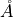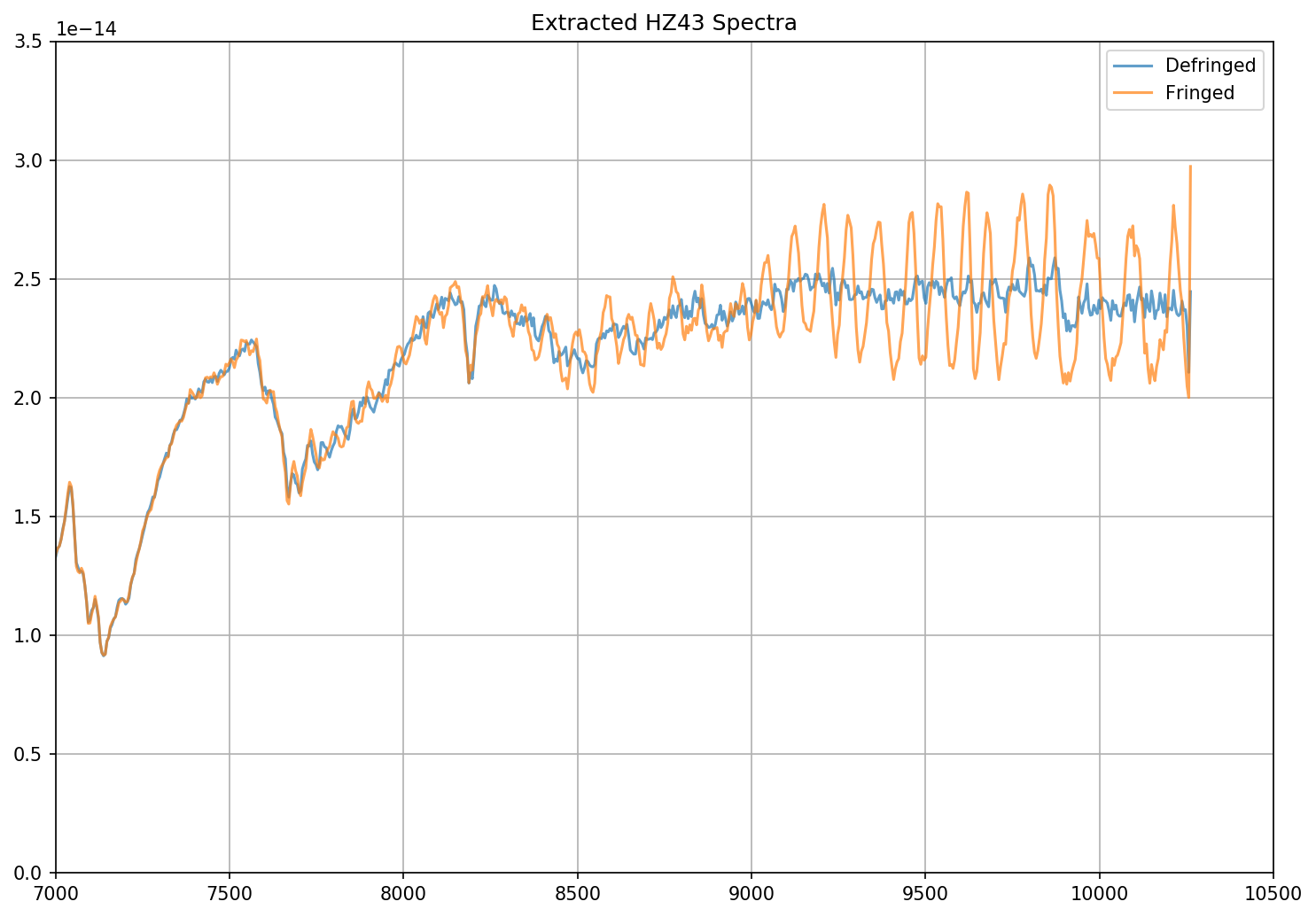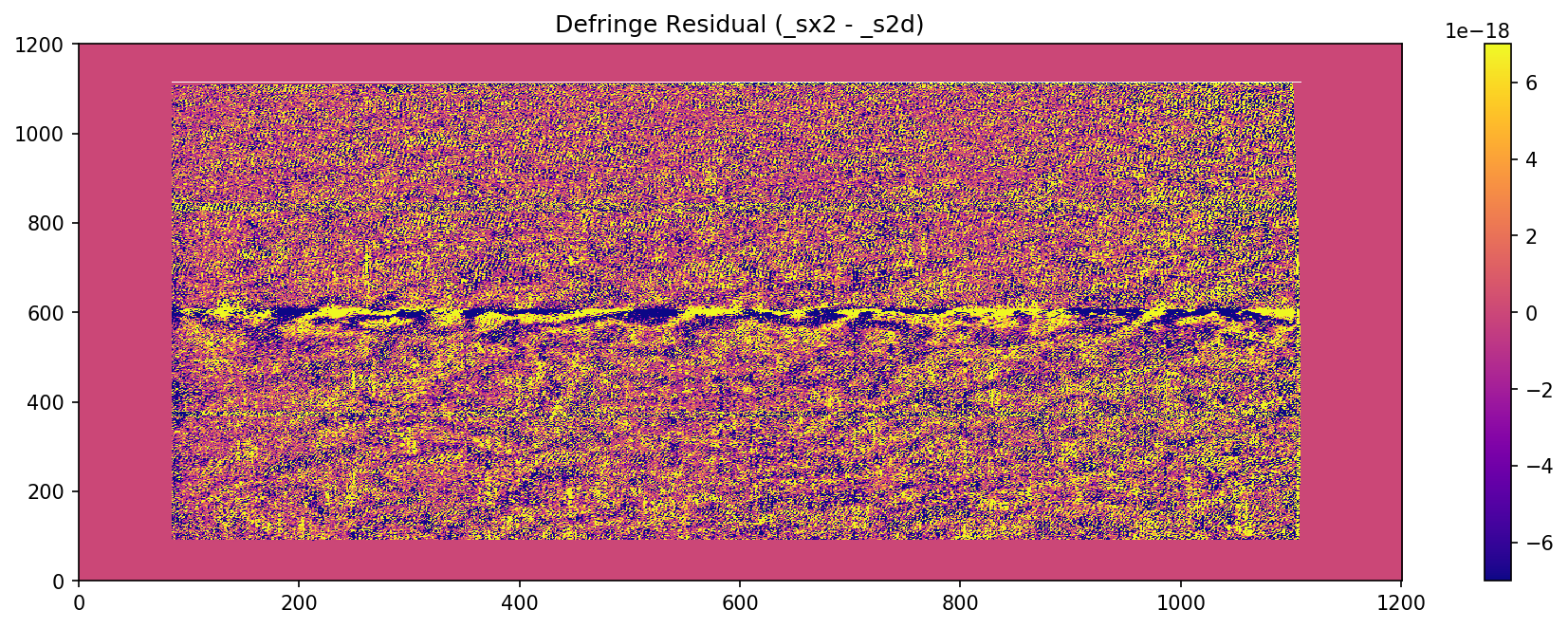```%matplotlib inline

import stistools
from astropy.io import fits
import matplotlib.pyplot as plt
import numpy as np
import glob
import matplotlib
```
```matplotlib.rcParams['image.origin'] = 'lower'
matplotlib.rcParams['image.aspect'] = 'auto'
matplotlib.rcParams['image.cmap'] = 'plasma'
matplotlib.rcParams['image.interpolation'] = 'none'
matplotlib.rcParams['figure.figsize'] = [15, 5]
```

# STIS CCD Defringing Examples¶

The Stistools defringing tools (`normspflat`,`prepspec`,`mkfringeflat`, and `defringe`) are needed to correct the fringing patterns that are present in the reddest STIS observing modes (>7000), namely G750M and G750L. The Defringing Guide provides a step-by-step tutorial on how to use these tools to perform this correction. The following serves to show several practical examples of it’s use on STIS data.

## G750L Observation of HZ43¶

```# Set up the data paths

sci_file = 'o49x18010'
flat_file = 'o49x18020'

# Normalize the contemoraneous flat field image
stistools.defringe.normspflat(f"{flat_file}_raw.fits",
f"{flat_file}_nsp.fits", do_cal=True,
wavecal=f"{sci_file}_wav.fits")
```
```File written:  /Users/stisuser/data/path/o49x18020_crj.fits
```
```# make the fringe flat, using the crj file for the science because this is G750L
stistools.defringe.mkfringeflat(f"{sci_file}_crj.fits", f"{flat_file}_nsp.fits",
f"{flat_file}_frr.fits")
```
```mkfringeflat.py version 0.1
- matching fringes in a flatfield to those in science data
Extraction center: row 511
Extraction size: 11.0 pixels  [Aperture: 52X2]
Range to be normalized: [506:517,4:1020]

Determining best shift for fringe flat

shift = -0.5, rms = 0.02521565587012733
shift = -0.4, rms = 0.02374587534351394
shift = -0.3, rms = 0.022735165926832522
shift = -0.19999999999999996, rms = 0.022235801562053452
shift = -0.09999999999999998, rms = 0.0223095350312966
shift = 0.0, rms = 0.022870072599462554
shift = 0.10000000000000009, rms = 0.023099186545412927
shift = 0.20000000000000007, rms = 0.0238499817578987
shift = 0.30000000000000004, rms = 0.025041375073110203
shift = 0.4, rms = 0.026649802114317847
shift = 0.5, rms = 0.02860146728021774

Best shift : -0.1963593679893798 pixels
Shifted flat : o49x18020_nsp_sh.fits
(Can be used as input flat for next iteration)

Determining best scaling of amplitude of fringes in flat

Fringes scaled  0.8: RMS = 0.022225777873537036
Fringes scaled  0.8400000000000001: RMS = 0.021245650237594484
Fringes scaled  0.88: RMS = 0.02073729355208867
Fringes scaled  0.92: RMS = 0.02073643145196044
Fringes scaled  0.9600000000000001: RMS = 0.021244380189719227
Fringes scaled  1.0: RMS = 0.022227696178223795
Fringes scaled  1.04: RMS = 0.023664059746596907
Fringes scaled  1.08: RMS = 0.025413279490181823
Fringes scaled  1.12: RMS = 0.02744748231768354
Fringes scaled  1.1600000000000001: RMS = 0.029710009708226032
Fringes scaled  1.2000000000000002: RMS = 0.03215458872298658

Best scale : 0.9182259885801685
Output flat : o49x18020_frr.fits
(to be used as input to task 'defringe.py')
```
```# Defringe the science spectrum
stistools.defringe.defringe(f"{sci_file}_crj.fits", f"{flat_file}_frr.fits", overwrite=True)
```
```Fringe flat data were read from the primary HDU
Imset 1 done
Defringed science saved to o49x18010_drj.fits
```
```'o49x18010_drj.fits'
```
```# Now, extract the spectra from both the fringed (crj) and defringed (drj) data
defringed = glob.glob(f"{sci_file}*drj.fits")
fringed = glob.glob(f"{sci_file}*crj.fits")
files = [defringed, fringed]

outnames = [f'{defringed.split("/")[-1].split("_")}_dx1d.fits', f'{fringed.split("/")[-1].split("_")}_x1d.fits']

for i in range(len(outnames)):
stistools.x1d.x1d(files[i], output=outnames[i])
```
```dx1d = fits.open(outnames)
x1d = fits.open(outnames)

# Plot both the fringed and the defringed 1D extracted spectra together
fig = plt.figure(figsize=(10,7),dpi=150)
plt.plot(dx1d.data['WAVELENGTH'], dx1d.data['FLUX'],'-', label='Defringed', alpha=0.7)
plt.plot(x1d.data['WAVELENGTH'], x1d.data['FLUX'],'-', label='Fringed', alpha=0.7)

plt.xlim(7000,10500)
plt.ylim(0, 3.5e-14)
plt.title("Extracted HZ43 Spectra")
plt.grid()
plt.legend()
plt.tight_layout()
```## G750M Observation of AGK+81D266¶

```#setup data paths

sci_file = "oe36m10g0"
flat_file = "oe36m10j0"

# Normalize the contemporaneous flat field image
stistools.defringe.normspflat(f"{flat_file}_raw.fits",
f"{flat_file}_nsp.fits", do_cal=True,
wavecal=f"{sci_file}_wav.fits")
```
```File written:  /Users/stisuser/data/path/oe36m10j0_sx2.fits
```
```/Users/stisuser/install/path/normspflat.py:216: RuntimeWarning: divide by zero encountered in true_divide
row_fit = fit_data/spl(xrange)
/Users/stisuser/install/path/normspflat.py:216: RuntimeWarning: invalid value encountered in true_divide
row_fit = fit_data/spl(xrange)
```
```# make the fringe flat, using the sx2 file for the science because this is G750M
stistools.defringe.mkfringeflat(f"{sci_file}_sx2.fits", f"{flat_file}_nsp.fits",
f"{flat_file}_frr.fits", beg_shift=-1.0, end_shift=0.5, shift_step=0.1,
beg_scale=0.8, end_scale=1.5, scale_step=0.04)
```
```mkfringeflat.py version 0.1
- matching fringes in a flatfield to those in science data
Extraction center: row 602
Extraction size: 11.0 pixels  [Aperture: 52X2]
Range to be normalized: [597:608,83:1106]

Determining best shift for fringe flat

shift = -1.0, rms = 0.06751209969416246
shift = -0.9, rms = 0.06750664734039523
shift = -0.8, rms = 0.06750325556538965
shift = -0.7, rms = 0.06750192457752245
shift = -0.6, rms = 0.06750265937240976
shift = -0.5, rms = 0.06750545682621624
shift = -0.3999999999999999, rms = 0.06750588470469428
shift = -0.29999999999999993, rms = 0.06751357970780972
shift = -0.19999999999999996, rms = 0.06752336035281563
shift = -0.09999999999999998, rms = 0.06753522897336409
shift = 0.0, rms = 0.06754918994601025
shift = 0.10000000000000009, rms = 0.06754304260847464
shift = 0.20000000000000018, rms = 0.0675389748466461
shift = 0.30000000000000004, rms = 0.06753698522431144
shift = 0.40000000000000013, rms = 0.06753708053436966
shift = 0.5, rms = 0.06753925625176614

Best shift : -0.6999982359381194 pixels
Shifted flat : oe36m10j0_nsp_sh.fits
(Can be used as input flat for next iteration)

Determining best scaling of amplitude of fringes in flat

Fringes scaled  0.8: RMS = 0.06762238400706264
Fringes scaled  0.8400000000000001: RMS = 0.06759376871355414
Fringes scaled  0.88: RMS = 0.06756741543477168
Fringes scaled  0.92: RMS = 0.0675433153759303
Fringes scaled  0.9600000000000001: RMS = 0.06752149090813866
Fringes scaled  1.0: RMS = 0.06750192634866954
Fringes scaled  1.04: RMS = 0.067484628741123
Fringes scaled  1.08: RMS = 0.06746960905640136
Fringes scaled  1.12: RMS = 0.06745685643406156
Fringes scaled  1.1600000000000001: RMS = 0.06744637965688902
Fringes scaled  1.2000000000000002: RMS = 0.06743817834085863
Fringes scaled  1.24: RMS = 0.06743225706483276
Fringes scaled  1.28: RMS = 0.06742860539788004
Fringes scaled  1.32: RMS = 0.06742723706558065
Fringes scaled  1.36: RMS = 0.06742814340085584
Fringes scaled  1.4: RMS = 0.06743132876996823
Fringes scaled  1.44: RMS = 0.06743679508124968
Fringes scaled  1.48: RMS = 0.06744453855109715
Fringes scaled  1.52: RMS = 0.06745456004413491

Best scale : 1.3200005501263359
Output flat : oe36m10j0_frr.fits
(to be used as input to task 'defringe.py')
```
```# defringe the science spectrum
stistools.defringe.defringe(f"{sci_file}_sx2.fits", f"{flat_file}_frr.fits", overwrite=True)
```
```Fringe flat data were read from the primary HDU
19 pixels in the fringe flat were less than or equal to 0
Imset 1 done
Defringed science saved to oe36m10g0_s2d.fits
```
```/Users/stisuser/install/path/defringe.py:95: RuntimeWarning: invalid value encountered in less_equal
```
```'oe36m10g0_s2d.fits'
```
```# Plot the fringe pattern removed from the sx2 file

scale = 7*10**-18
resid = fits.getdata(f"{sci_file}_sx2.fits")-fits.getdata(f"{sci_file}_s2d.fits")

fig = plt.figure(dpi=150)
plt.imshow(resid,vmin=-scale, vmax=scale)
plt.title("Defringe Residual (_sx2 - _s2d)")

cbar = plt.colorbar()
```## Subarray Data¶

If the science data is subarray data, there are a few extra steps in the process before defringing.

```# Set up the data paths
sci_file = 'odqf11trq'
flat_file = 'odqf11060'
```
```# Normalize the contemporaneous flat field image
stistools.defringe.normspflat(f"{flat_file}_raw.fits",
f"{flat_file}_nsp.fits", do_cal=True,
wavecal=f"{sci_file}_wav.fits")
```
```File written:  /Users/stisuser/data/path/odqf11060_crj.fits
```

If using G750L data, set the order sorter fringes in the flat field equal to one. This particular dataset is G750L data.

```with fits.open(f"{flat_file}_nsp.fits", mode="update") as flat_hdu:
flat_data = flat_hdu.data
flat_data[:, :250] = 1
```

Next, pad the subarray data with zeros so that it is the same size as the flat field data

```sci = fits.open(f"{sci_file}_crj.fits")
sci_data = sci.data
flat_data = fits.getdata(f"{flat_file}_nsp.fits")

full_sci = np.zeros(np.shape(flat_data), dtype='float32')
full_sci = [int(ymin_sci):int(ymax_sci), :] = sci_data
full_dq = np.zeros(np.shape(flat_data), dtype='int16')
full_dq[int(ymin_sci):int(ymax_sci), :] = sci_dq
full_err = np.zeros(np.shape(flat_data), dtype='float32')
full_err[int(ymin_sci):int(ymax_sci), :] = sci_err
```

Put the full frame data into a new file and populate the headers

```header1 = sci.header

# Create the new fits file
hdu = fits.HDUList([empty_primary, image_hdu, err_hdu, dq_hdu])

fullframe_output = f"{sci_file}_fullfield_crj.fits"
hdu.writeto(fullframe_output)
```

Finally, change the LTV2 header keyword to zero

```fits.setval(fullframe_output, 'LTV2', ext=1, value=0.0)
```

Make the fringe flat by running the newly created full-frame image through mkfringeflat

```stistools.defringe.mkfringeflat(fullframe_output, f"{flat_file}_nsp.fits",
f"{flat_file}_frr.fits")
```
```mkfringeflat.py version 0.1
- matching fringes in a flatfield to those in science data
Extraction center: row 497
Extraction size: 11.0 pixels  [Aperture: 52X2]
Range to be normalized: [492:503,4:1020]

Determining best shift for fringe flat

shift =     -0.500, rms =   0.0163
shift =     -0.400, rms =   0.0152
shift =     -0.300, rms =   0.0145
shift =     -0.200, rms =   0.0145
shift =     -0.100, rms =   0.0149
shift =      0.000, rms =   0.0159
shift =      0.100, rms =   0.0165
shift =      0.200, rms =   0.0175
shift =      0.300, rms =   0.0189
shift =      0.400, rms =   0.0206
shift =      0.500, rms =   0.0225

Best shift :     -0.205 pixels
Shifted flat : odqf11060_nsp_sh.fits
(Can be used as input flat for next iteration)

Determining best scaling of amplitude of fringes in flat

Fringes scaled       0.800: RMS =   0.0125
Fringes scaled       0.840: RMS =   0.0117
Fringes scaled       0.880: RMS =   0.0115
Fringes scaled       0.920: RMS =   0.0120
Fringes scaled       0.960: RMS =   0.0130
Fringes scaled       1.000: RMS =   0.0145
Fringes scaled       1.040: RMS =   0.0162
Fringes scaled       1.080: RMS =   0.0182
Fringes scaled       1.120: RMS =   0.0204
Fringes scaled       1.160: RMS =   0.0227
Fringes scaled       1.200: RMS =   0.0251

Best scale :      0.878
Output flat : odqf11060_frr.fits
(to be used as input to task 'defringe.py')
```

Finally, defringe the data as usual.

```stistools.defringe.defringe(fullframe_output, f"{flat_file}_frr.fits", overwrite=True)
```
```Fringe flat data were read from the primary HDU
4 pixels in the fringe flat were less than or equal to 0
Imset 1 done
Defringed science saved to odqf11trq_fullfield_drj.fits
```